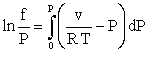Contents - Index

FUGACITY

The FUGACITY function returns the fugacity of the specified pure fluid for the given conditions. Fugacity is defined by the following relation:where

f  is fugacity (same units as pressure)

P is pressure

v is the specific volume of the fluid

T is the absolute temperature

R is the gas constant.

For an ideal gas fluid, f is identical to P.

The FUGACITY function will accept any appropriate set of inputs.  Fugacity is particularly useful for some types of phase equilibrium calculations.

Example:

f=FUGACITY (R\$,T=100,P=101.3)

Return to Thermophysical Functions